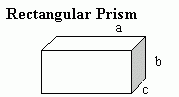Email us to get an instant 20% discount on highly effective K-12 Math & English kwizNET Programs!

#### Online Quiz (WorksheetABCD)

Questions Per Quiz = 2 4 6 8 10

### Grade 5 - Mathematics11.7 Rectangular Prisms and Cubes; and Volumes - Review Test

 Q 1: What is the name of the shape?heptagonal pyramidtriangular pyramidoctagonal pyramid Q 2: What is the name of the shape shown.Square PrismRectangular PrismCube Q 3: What is the name of this shape?heptagonal pyramidoctagonal pyramidtriangular pyramid Q 4: Find the height of the cylinder given that the circumference of the circular base is 12.56 and are 144 square units.Answer: Q 5: What is a special kind of rectangular prism with all its edges having same length and all its faces congruent.solidcubesphere Q 6: A polyhedron must be made up of the same shaped face.TrueFalse Q 7: The volume of a cube with side length 2 cm is ____ cm3.Answer: Q 8: A can of paint holds enough paint for 20 square meters. How many cans of paint do you need to paint a wall of height 5 m and length 8 m?8125 Question 9: This question is available to subscribers only! Question 10: This question is available to subscribers only!